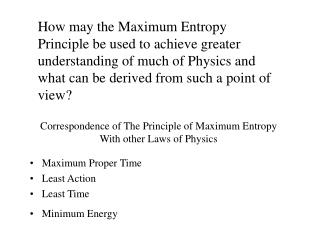Download PresentationCorrespondence of The Principle of Maximum Entropy With other Laws of Physics

Loading in 2 Seconds...

# Correspondence of The Principle of Maximum Entropy With other Laws of Physics - PowerPoint PPT Presentation

How may the Maximum Entropy Principle be used to achieve greater understanding of much of Physics and what can be derived from such a point of view?. Correspondence of The Principle of Maximum Entropy With other Laws of Physics. Maximum Proper Time Least Action Least Time Minimum Energy.I am the owner, or an agent authorized to act on behalf of the owner, of the copyrighted work described.
Download Presentation## Correspondence of The Principle of Maximum Entropy With other Laws of Physics

An Image/Link below is provided (as is) to download presentation

Download Policy: Content on the Website is provided to you AS IS for your information and personal use and may not be sold / licensed / shared on other websites without getting consent from its author.While downloading, if for some reason you are not able to download a presentation, the publisher may have deleted the file from their server.

- - - - - - - - - - - - - - - - - - - - - - - - - - E N D - - - - - - - - - - - - - - - - - - - - - - - - - -
Presentation Transcript
1. How may the Maximum Entropy Principle be used to achieve greater understanding of much of Physics and what can be derived from such a point of view? Correspondence of The Principle of Maximum Entropy With other Laws of Physics • Maximum Proper Time • Least Action • Least Time • Minimum Energy

2. In a relativistic universe Atomic Clocks’ events appear at differing rates depending on the world line of the frame of reference • It’s the comparative rates of events that change in our relativistic universe • For the following discussion I would like to use the concept of “EVENTS” to replace TIME • The TIC of the clock is the atomic events E1,E2,E3…… What has been done is to replace the viewpoint of time with a viewpoint of Events to see what arises as a result

3. Principle of Maximum Proper Time • A body in motion takes the path in space-time that maximizes the passage of proper time according to its own built in “WRISTWATCH” aka “EVENTS” E1,E2…. This will maximize the Entropic Rate • This principle is valid on a differential scale also • An example is a free falling body that “senses” no force …Proper time is maximized by this condition By treating Proper time as a dimension where entropy is maximized by virtue of Proper time being maximum one can attain a new viewpoint and solve problems

4. Contrast Free Fall with static position in Gravitational Field • At rest on ground (accelerated) In Free - Fall • Ent [Exponential Distribution] < Ent [Uniform Distribution] (Ent= entropy of operator) • Spatial entropy is maximized in both depicted conditions • Its much easier to see why inertial mass = gravitational mass under this viewpoint

5. The Principle of Least Action can be derived from the principle of Maximum Proper time • Least Action also maximizes the space-time for events and therefore maximizes entropy …so it appears that max entropy principle extends from space and time to spacetime • This principle works on the differential scale also • d ( action ) = K.E. -P.E. ….this maximizes the P.E. and therefore the proper time passage is greatest • F = M*A can be derived from Least Action Principle • Feynman derived Schrodingers Wave Equation using the Principle of Least Action as a starting point

6. Schrodinger’s Equation yields Maximum Entropy solutions • This answers a question that I have had for a long time • Question: Why is it valid to use a sinusoidal waveform when modeling physical systems such as atoms in a lattice or electrons in an antenna? • Answer: For a particle constrained to a given energy and position over interval [ - inf , + inf ] the maximum entropy wave function is gaussian in the base state • HARMONIC POTENTIAL =>sinusoidal!!

7. Correspondence of Least Energy and Maximum Entropy on the Micro Scale • As a particle is confined more it’s energy goes up • Since Schrodinger’s maximizes wavefunction entropy the energy level is minimized for a given confinement • This is analogous to a gas in a box

8. Principle of Least Time • A photon knows no time because it travels at the speed of light…. • Emission and absorption appear to occur simultaneously from the point of view of the photon • From the Universe around the photon Entropic rate will be maximized if the light takes the least time between emission and absorption as measured by those of us with rest mass that experience “time”

9. Entropic Theory of Gravitation • Above newtons law of gravity is derived by differentiating proper time with respect to distance and substituting for the Schwarzchild radius (with a correction factor !) • One can see that the force of gravity on an object is an entropic pressure … in free fall an object wants to “HOP” to the most likely next state and does so • The correction factor shows the force to be infinite at the event horizon….

10. Results • As a result of this investigation I have been able to see that the physical bodies experience some sort of entropic pressure that governs their motion……A modified Newton’s theory of gravitation may be derived • In place of time I substituted a progression of events in which entropy in its enumeration of physical states and seems to be much more useful in some fundamental way • Maximum entropy method applies to space and time similtaneously